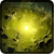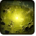# Acid BlastConditions

## Related effects, buffs and debuffs

Please click on an effect below to view its details.

• [hidden] [600s]Effect 4367801351405840

 Slot: Buff Duration: 10m 0s Tick rate: does not tick # occurrences: 0 Tags: 8841933366126930507 Conditions: If difficulty is set to Story Mode Target overrides: [target override] Aoe Sphere
• On Apply

Perform the following actions:

• Unknown (183)
- Unknown (303) = (bool) true
- Unknown (306) = (bool) true
- Unknown (430) = (bool) false
- Unknown (310) = (string) aoePositionOptionType_Entity
- Unknown (311) = (string) aoeTarget_PrimaryTarget
- Unknown (313) = (string) aoeScalarType_LiteralValue
- Unknown (317) = (string) aoeScalarType_LiteralValue
- Unknown (321) = (string) aoeScalarType_LiteralValue
- Unknown (325) = (string) aoeOrientationOptionType_WorldCoordinates
- Unknown (344) = (string) aoeSphere
- Unknown (349) = (string) aoeTargetCondition_IsPlayer;aoeTargetCondition_IsAlive
- Unknown (353) = (string) aoeScalarType_LiteralValue
- Unknown (354) = (string) aoeScalarType_LiteralValue
- Unknown (355) = (string) aoeScalarType_LiteralValue
- Unknown (367) = (string) aoeScalarType_LiteralValue
- Unknown (384) = (string)
- Unknown (386) = (string) aoeAbilityCaster_Caster
- Unknown (387) = (string) aoeAbilityTarget_Target
- Unknown (388) = (string)
- Unknown (389) = (string)
- Unknown (431) = (string) aoeRotation_None
- Unknown (551) = (string) aoeVfx_None
- Unknown (552) = (string) aoeVfx_None
- Effect Number = (int) 0
- Ability Spec = (int) 16140978188393207287
- Unknown (307) = (int) 150
- Unknown (308) = (int) 300
- Tick Rate = (float) 2
- Unknown (304) = (float) 300
- Unknown (305) = (float) 600
- Unknown (314) = (float) 0
- Unknown (318) = (float) 0
- Unknown (322) = (float) 0
- Unknown (362) = (float) 0
- Unknown (363) = (float) 0
- Unknown (364) = (float) 0
- Unknown (368) = (float) 1.1
- Unknown (390) = (float) 0
- Unknown (398) = (float) 0.1
- Unknown (616) = (float) 1
- Unknown (617) = (float) 1
- Unknown (618) = (float) 1
- Unknown (623) = (float) 1
- Unknown (624) = (float) 1
- Unknown (625) = (float) 1
• Play appearance epp . operation . dxun . boss . hybrid_horror . hybrid_horror . acid_blast_ground_puddle_story, dependent on calling effect
• [hidden] [600s]Effect 4367801351405845

 Slot: Buff Duration: 10m 0s Tick rate: does not tick # occurrences: 0 Tags: 8841933366126930507 Conditions: If difficulty is set to Hard or Nightmare Mode Target overrides: [target override] Aoe Sphere
• On Apply

Perform the following actions:

• Unknown (183)
- Unknown (303) = (bool) true
- Unknown (306) = (bool) true
- Unknown (430) = (bool) false
- Unknown (584) = (bool) false
- Unknown (310) = (string) aoePositionOptionType_Entity
- Unknown (311) = (string) aoeTarget_PrimaryTarget
- Unknown (313) = (string) aoeScalarType_LiteralValue
- Unknown (317) = (string) aoeScalarType_LiteralValue
- Unknown (321) = (string) aoeScalarType_LiteralValue
- Unknown (325) = (string) aoeOrientationOptionType_WorldCoordinates
- Unknown (344) = (string) aoeSphere
- Unknown (349) = (string) aoeTargetCondition_IsPlayer;aoeTargetCondition_IsAlive
- Unknown (353) = (string) aoeScalarType_LiteralValue
- Unknown (354) = (string) aoeScalarType_LiteralValue
- Unknown (355) = (string) aoeScalarType_LiteralValue
- Unknown (367) = (string) aoeScalarType_LiteralValue
- Unknown (384) = (string)
- Unknown (386) = (string) aoeAbilityCaster_Caster
- Unknown (387) = (string) aoeAbilityTarget_Target
- Unknown (388) = (string)
- Unknown (389) = (string)
- Unknown (431) = (string) aoeRotation_None
- Unknown (534) = (string) aoeScaling_FixedAmount
- Unknown (551) = (string) aoeVfx_None
- Unknown (552) = (string) aoeVfx_None
- Unknown (587) = (string) aoeScaling_StopScaling
- Effect Number = (int) 0
- Ability Spec = (int) 16140978188393207287
- Unknown (307) = (int) 150
- Unknown (308) = (int) 300
- Tick Rate = (float) 2
- Unknown (304) = (float) 300
- Unknown (305) = (float) 600
- Unknown (314) = (float) 0
- Unknown (318) = (float) 0
- Unknown (322) = (float) 0
- Unknown (362) = (float) 0
- Unknown (363) = (float) 0
- Unknown (364) = (float) 0
- Unknown (368) = (float) 1.4
- Unknown (390) = (float) 0
- Unknown (398) = (float) 0.1
- Unknown (582) = (float) 4.4
- Unknown (592) = (float) 0.01
- Unknown (616) = (float) 1
- Unknown (617) = (float) 1
- Unknown (618) = (float) 1
- Unknown (623) = (float) 1
- Unknown (624) = (float) 1
- Unknown (625) = (float) 1
• Play appearance epp . operation . dxun . boss . hybrid_horror . hybrid_horror . acid_blast_ground_puddle_expanding, dependent on calling effect